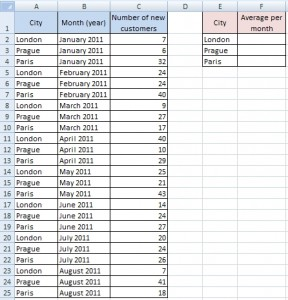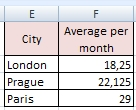# Average with condition

There is a problem with average for some specific value in a table. Following table represents data of some worldwide company. You can see branch offices in the cities and number of their new customers per each month.How to do average for specific items (London, Prague and Paris)?

## Solution for Excel 2007

For version 2007 is very easy to calculate this. Just use AVERAGEIF function. So, for London is formula like this:
`=AVERAGEIF(A2:A25;E2;C2:C25)`
If you need to copy this to Prague and Paris, you have to fill \$ character into the formula. Be careful, \$ isn’t in each argument.
`=AVERAGEIF(\$A\$2:\$A\$25;E2;\$C\$2:\$C\$25)`

## Solution for Excel 2003

In version 2003 is AVERAGEIF function missing. Therefore we have to use array formula:
`{=AVERAGE(IF(A2:A25=E2,C2:C25))}`
As in the previous example, If you need to copy this to Prague and Paris, you have to fill \$ character into the formula.
`{=AVERAGE(IF(\$A\$2:\$A\$25=E2,\$C\$2:\$C\$25))}`